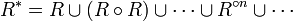# Transitive relation

In mathematics, a transitive relation on a set is a relation with the property that if xy and yz then xz.

## Examples

• An equivalence relation is transitive:
• Equality is transitive: if x=y and y=z then x=z;
• The trivial (always-true) relation is transitive;
• An order relation is transitive:
• The usual order on the integers is transitive: if x>y and y>z then x>z;
• Divisibility on the natural numbers is transitive: if x divides y and y divides z then x divides z;
• Inclusion on subsets of a set is transitive: if x is a subset of y and y is a subset of z then x is a subset of z.

## Properties

• The intersection of transitive relations is transitive. That is, if R and S are transitive relations on a set X, then the relation R&S, defined by ( x R&S y ) if ( x R y and x S y ), is also transitive. The same holds for intersections of arbitrary families of transitive relations: indeed, the transitive relations on a set form a closure system.

Transitivity may be defined in terms of relation composition. A relation R is transitive if the composite R.R implies (is contained in) R.

## Transitive closure

The transitive closure of a relation R may be defined as the intersection R* of all transitive relations containing R (one always exists, namely the always-true relation): loosely the "smallest" transitive relation containing R. The closure may also be constructed as$R^* = R \cup (R\circ R) \cup \cdots \cup R^{{\circ}n} \cup \cdots \,$

where$R^{{\circ}n}$ denotes the composition of R with itself n times.Some content on this page may previously have appeared on Citizendium.